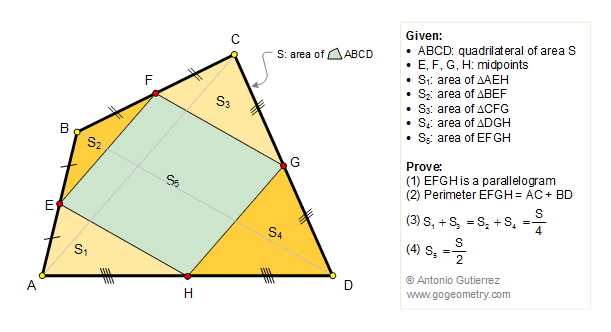# Problem 146. Varignon's Theorem: Quadrilateral, Midpoints, Parallelogram, Area, Perimeter.

 In the figure below, ABCD is a quadrilateral of area S. E, F, G, and H are the midpoints of the sides. S1, S2, S3, and S4 are the areas of triangles AEH, BEF, CFG, and DGH respectively. Prove that: (1) EFGH is a parallelogram, called Varignon parallelogram, (2) the perimeter of the Varignon parallelogram is equal to the sum of diagonals of ABCD, (3) S1+ S3 = S2 + S4 = S / 4, (4) the area of the Varignon parallelogram is half that of ABCD.Home | Geometry | Search | Problems | Art | 141-150 | Semiperimeter | Area of a Parallelogram | Art of Varignon's theorem | Email | View or post a solution | by Antonio Gutierrez